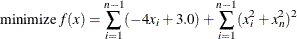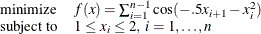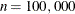### Example 8.2 Solving Unconstrained and Bound-Constrained Optimization Problems

Although the NLP techniques are suited for solving generally constrained nonlinear optimization problems, these techniques can also be used to solve unconstrained and bound-constrained problems efficiently. This example considers the relatively large nonlinear optimization problemsandwith. These problems are unconstrained and bound-constrained, respectively.

For large-scale problems, the default memory limit might be too small, which can lead to out-of-memory status. To prevent this occurrence, it is recommended that you set a larger memory size. See the section Memory Limit for more information.

To solve the first problem, you can write the following statements:

proc optmodel;
number N=100000;
var x{1..N} init 1.0;

minimize f = sum {i in 1..N - 1} (-4 * x[i] + 3.0)  +
sum {i in 1..N - 1} (x[i]^2 + x[N]^2)^2;

solve with nlp;
quit;


The problem and solution summaries are shown in Output 8.2.1.

Output 8.2.1: Problem Summary and Solution Summary

The OPTMODEL Procedure

Problem Summary
Objective Sense Minimization
Objective Function f
Objective Type Nonlinear

Number of Variables 100000
Bounded Above 0
Bounded Below 0
Bounded Below and Above 0
Free 100000
Fixed 0

Number of Constraints 0

Performance Information
Execution Mode Single-Machine

Solution Summary
Solver NLP
Algorithm Interior Point
Objective Function f
Solution Status Optimal
Objective Value 0

Optimality Error 1.007903E-14
Infeasibility 0

Iterations 16
Presolve Time 0.02
Solution Time 15.03

To solve the second problem, you can write the following statements (here the active-set method is specifically selected):

proc optmodel;
number N=100000;
var x{1..N} >= 1 <= 2;

minimize f = sum {i in 1..N - 1} cos(-0.5*x[i+1] - x[i]^2);

solve with nlp / algorithm=activeset;
quit;


The problem and solution summaries are shown in Output 8.2.2.

Output 8.2.2: Problem Summary and Solution Summary

The OPTMODEL Procedure

Problem Summary
Objective Sense Minimization
Objective Function f
Objective Type Nonlinear

Number of Variables 100000
Bounded Above 0
Bounded Below 0
Bounded Below and Above 100000
Free 0
Fixed 0

Number of Constraints 0

Performance Information
Execution Mode Single-Machine

Solution Summary
Solver NLP
Algorithm Active Set
Objective Function f
Solution Status Optimal
Objective Value -99999

Optimality Error 1.437473E-12
Infeasibility 0

Iterations 8
Presolve Time 0.02
Solution Time 18.38